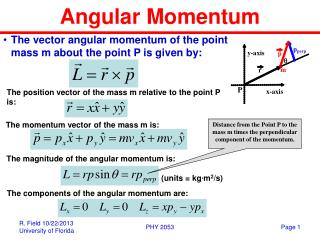DownloadDownload PresentationAngular Momentum

# Angular Momentum

Télécharger la présentation## Angular Momentum

- - - - - - - - - - - - - - - - - - - - - - - - - - - E N D - - - - - - - - - - - - - - - - - - - - - - - - - - -
##### Presentation Transcript

1. Angular Momentum • The vector angular momentum of the point mass m about the point P is given by: The position vector of the mass m relative to the point P is: The momentum vector of the mass m is: Distance from the Point P to the mass m times the perpendicular component of the momentum. The magnitude of the angular momentum is: (units = kg∙m2/s) The components of the angular momentum are: PHY 2053

2. Torque • The torque vector about the point P due to the force F acting at r is given by: The position vector of the mass m relative to the point P is: The force acting on the mass m is: Distance from the Point P to the mass m times the perpendicular component of the force. The magnitude of the torque is: (units = N∙m) The components of the torque are: PHY 2053

3. Rotation: Angular Variables • Arc Length: The arc length s is related to the angle q (in radians = rad) as follows: (360o = 2p rad) • Angular Displacement and Angular Velocity: (radians/second) • Tangential Velocity and Angular Velocity: Tangential Velocity • Angular Acceleration: (radians/s2) PHY 2053

4. Rolling Without Slipping:Rotation & Translation • If a cylinder of radius R rolls without slipping along the x-axis then: Translational Speed Rotational Speed PHY 2053

5. Translation vs Rotation • Translation: • Rotation: Mass: m Moment of Inertia: I Position: x Angular Position: q Velocity: vx Angular Velocity: w Acceleration: ax Angular Acceleration: a Force: Torque: constant then If constant If then Angular Momentum Conservation! Momentum Conservation! PHY 2053

6. Exam 2 Fall 2010: Problem 11 • A non-uniform cylinder with mass M and radius R rolls without sliding along the floor. If its translational kinetic energy is three times greater than its rotational kinetic energy about the rotation axis through its center of mass (i.e. the central axis of the cylinder), what is its moment of inertia about the central axis? Answer: MR2/3 % Right: 44% PHY 2053

7. Example: Rolling without Slipping • If a cylinder with moment of inertia I and radius R starts from rest at a height h above the ground and rolls without slipping down an incline. What is its translational speed when it reaches the ground? • Example: I = MR2/2 (solid cylinder), h = 9.8 m then PHY 2053

8. Example: Rolling without Slipping • If a cylinder with moment of inertia I and radius R starts from rest at a height h above the ground and rolls without slipping down an incline. If the cylinder starts from rest at t = 0, when does it reach the ground? • Example: I = MR2/2 (solid cylinder), h = 9.8 m, q = 45o then PHY 2053

9. Exam 2 Fall 2010: Problem 14 • A 100-N uniform plank leans against a frictionless wall as shown. What is the magnitude of the torque (about the point P) applied to the plank by the wall? Answer: 150 N∙m % Right: 42% PHY 2053

10. Exam 2 Fall 2011: Problem 13 • A thin stick with mass M, length L, and moment of inertia ML2/3 is hinged at its lower end and allowed to fall freely as shown in the figure. If its length L = 2 m and it starts from rest at an angle q = 20o, what is the speed (in m/s) of the free end of the stick when it hits the table? Answer: 7.43 m/s % Right: 14% PHY 2053